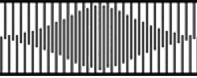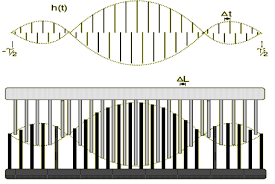#### Howdy, Stranger!

It looks like you're new here. If you want to get involved, click one of these buttons!

# Sine wave shape

Hello guys,
I need to code this shape with python script, i already created the code to insert the fingers(paths) but they all the same lenght, i need an idea to make it as a sine wave like this.
If you have any idea i would be thankful.• Please paste the code you have. I cannot help you with this otherwise.

Matthias

• Dear Matthias,
soory for not posting the code.
i will post it here in the commetns

• ``````for n in range(int(self.nIDT/2)):

#right finger first part
startPoint = pya.Point(-100, ((widthIDT/2 + (2*n)*pitchIDT+distanceSAW)*1000))
endPoint = pya.Point( ((L2)*1000), ((widthIDT/2 + (2*n)*pitchIDT+distanceSAW)*1000))
self.cell.shapes(self.l_layer).insert(pya.Path([startPoint,endPoint], widthIDT*1000, 0, 0))

startPoint = pya.Point(-100,((5*widthIDT/2 + (2*n)*pitchIDT+distanceSAW)*1000))
endPoint = pya.Point(((L2)*1000), ((5*widthIDT/2 + (2*n)*pitchIDT+distanceSAW)*1000))
self.cell.shapes(self.l_layer).insert(pya.Path([startPoint,endPoint], widthIDT*1000, 0, 0))

#right finger second part
startPoint = pya.Point((L2+self.lambdaIDT)*1000, ((widthIDT/2 + (2*n)*pitchIDT+distanceSAW)*1000))
endPoint = pya.Point( ((wzero)*1000)+100, ((widthIDT/2 + (2*n)*pitchIDT+distanceSAW)*1000))
self.cell.shapes(self.l_layer).insert(pya.Path([startPoint,endPoint], widthIDT*1000, 0, 0))

startPoint = pya.Point((L2+self.lambdaIDT)*1000, ((5*widthIDT/2 + (2*n)*pitchIDT+distanceSAW)*1000))
endPoint = pya.Point( ((wzero)*1000)+100, ((5*widthIDT/2 + (2*n)*pitchIDT+distanceSAW)*1000))
self.cell.shapes(self.l_layer).insert(pya.Path([startPoint,endPoint], widthIDT*1000, 0, 0))

#left finger first part
startPoint = pya.Point(-100, ((widthIDT/2 + (2*n+1)*pitchIDT+distanceSAW)*1000))
endPoint = pya.Point( ((w)*1000), ((widthIDT/2 + (2*n+1)*pitchIDT+distanceSAW)*1000))
self.cell.shapes(self.l_layer).insert(pya.Path([startPoint,endPoint], widthIDT*1000, 0, 0))

startPoint = pya.Point(-100, ((5*widthIDT/2 + (2*n+1)*pitchIDT+distanceSAW)*1000))
endPoint = pya.Point( ((w)*1000), ((5*widthIDT/2 + (2*n+1)*pitchIDT+distanceSAW)*1000))
self.cell.shapes(self.l_layer).insert(pya.Path([startPoint,endPoint], widthIDT*1000, 0, 0))

#left finger second part
startPoint = pya.Point((w+self.lambdaIDT)*1000, ((widthIDT/2 + (2*n+1)*pitchIDT+distanceSAW)*1000))
endPoint = pya.Point(((wzero)*1000)+100, ((widthIDT/2 + (2*n+1)*pitchIDT+distanceSAW)*1000))
self.cell.shapes(self.l_layer).insert(pya.Path([startPoint,endPoint], widthIDT*1000, 0, 0))

startPoint = pya.Point((w+self.lambdaIDT)*1000, ((5*widthIDT/2 + (2*n+1)*pitchIDT+distanceSAW)*1000))
endPoint = pya.Point( ((wzero)*1000)+100, ((5*widthIDT/2 + (2*n+1)*pitchIDT+distanceSAW)*1000))
self.cell.shapes(self.l_layer).insert(pya.Path([startPoint,endPoint], widthIDT*1000, 0, 0))
``````
• I don't see a sine or cosine function. Am I missing something?

Matthias

•dear Matthias,
with my code i am currently getting these shape

•and i want a sine function to get something like this

• Hi, Abdullah_Altayara,
Excuse me, but what is the "self" in your code?

• Hi Abdullah,

I'm sorry, but I don't think I can help here.

The function you depict is not a sine, it's rather "sin(x)/x" or something like this. Plus, your code is just partial, so it's lacking the interesting details. It's rather tedious to guess anyone's intention from the code with such little information.

But worst of all, I don't see any indication of an attempt to solve the problem. Even without knowing the details about L2, w, wzero, nIDT, lambdaIDT etc. it's not hard to tell that this code will never produce anything sine-like - simply because there is no function call of "sin" or even "cos". So of course the pattern doesn't look like the one you want to have.

But programming is not about wishing something and then asking someone else to implement it.

Matthias